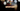# Leetcode | Solution of First Unique Character in a String in JavaScript

May 13th, 2020
|

In this post, we will solve First Unique Character in a String from leetcode and compute it's time and space complexities. Let's begin.

# Problem Statement

The question can be found at leetcode First Unique Character in a String problem.

The problem states that we are given a string, and we need to find the first character which is unique in the string

# Solution

One simple approach to solve this is to pick each character iteratively and search it in the string. If it is not found at any index then we have found our answer. This, however, will be of quadratic time complexity. We can do better at the expense of extra space.

We can have a map, and iteratively save each character with its index in the map. While adding to map, if we find that number already exists in the map, we save its index as -1(just to denote that it has duplicates). Once we are done with our string, the first element in the map to have a non-negative index is our first unique character.

We have discussed the approach, I urge you to go ahead on leetcode and give it another try.If you are here, it means something went wrong in implementation or you are just too lazy. In any case, let's see a simple implementation of the above logic.
``````var firstUniqChar = function (s) {
const map = new Map();

for (let i = 0; i < s.length; i++) {
if (map.has(s[i])) {
map.set(s[i], -1)
} else {
map.set(s[i], i)
}
}
for (let [key, value] of map) {
if (value !== -1) {
return value;
}
}

return -1;
};``````

Let's look at the solution. Now we are looping over the string only once and marking the duplicate ones. When we are done with the loop, we have the characters which are unique with their indices.

Here are the stats on submission

``````Status: Accepted
Runtime: 96ms
Memory: 37.9MB``````

## Time and space complexity

### Time complexity

We are looping over the string once, so time complexity would be O(n).

### Space complexity

We are using extra space for a map. So space complexity is linear, O(n).

# Summary

So, we solved the First Unique Character in a String problem by using a map and calculated the time and space complexities.

I hope you enjoyed solving this question. This is it for this one, complete source code for this post can be found on my Github Repo. Will see you in the next one.